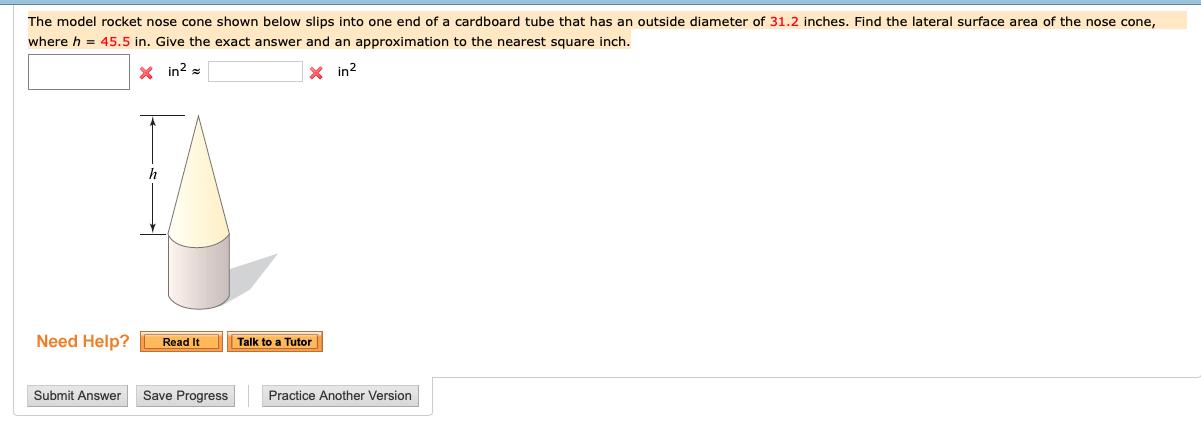The model rocket nose cone shown below slips into one end of a cardboard tube that has an outside diameter of 31.2 inches. Find the lateral surface area of the nose cone,where h = 45.5 in. Give the exact answer and an approximation to the nearest square inchX in2Need Help? Read ItTalk to a TutorSubmit AnswerSave ProgressPractice Another Version

Question

The model rocket nose cone shown below slips into one end of a cardboard tube that has an outside diameter of 31.2 inches. Find the lateral surface area of the nose cone, where h = 45.5 in. Give the exact answer and an approximation to the nearest square inch.help_outlineImage TranscriptioncloseThe model rocket nose cone shown below slips into one end of a cardboard tube that has an outside diameter of 31.2 inches. Find the lateral surface area of the nose cone, where h = 45.5 in. Give the exact answer and an approximation to the nearest square inch X in2 Need Help? Read It Talk to a Tutor Submit Answer Save Progress Practice Another Version fullscreen
Step 1

The diameter of the nose cone is 31.2 inches and height is 45.5 inches.

Lateral surface of a cone is πrl square units.

Obtain the radius of the cone.

Step 2

Obtain the slant height of the cone.

Step 3

Now substitutes the value of r and l in the l...

Want to see the full answer?

See Solution

Want to see this answer and more?

Our solutions are written by experts, many with advanced degrees, and available 24/7

See Solution
Tagged in

Geometry